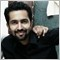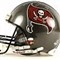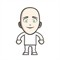# Indicators with alerts/signal - page 1489201222

mony:
sir can you please do this if possible

For start, check this thread : https://www.mql5.com/en/forum/172894 - I am quite sure that there are at least a couple of pivot indicators there that can do that already85

Is it possible to get arrows and alert when the crossover occurs as mentioned in the snapshot.

I have also attached the required indicators.

Thanks as always :)

Files:
snapshot.JPG 114 kb
RSI.mq4 5 kb
RSI.ex4 11 kb520763

study(title="Double HullMA Cross", overlay=true)

n=input(title="period",type=integer,defval=7)

n2ma=2*wma(close,round(n/2))

nma=wma(close,n)

diff=n2ma-nma

sqn=round(sqrt(n))

n2ma1=2*wma(close,round(n/2))

nma1=wma(close,n)

diff1=n2ma1-nma1

sqn1=round(sqrt(n))

n1=wma(diff,sqn)

n2=wma(diff1,sqn)

c=n1>n2?lime:red

plot(n1,color=c, linewidth = 1)

plot(n2,color=c, linewidth = 1)

plot(cross(n1, n2) ? n1 : na, style = cross, color = c, linewidth = 4)626

pacois:

study(title="Double HullMA Cross", overlay=true)

n=input(title="period",type=integer,defval=7)

n2ma=2*wma(close,round(n/2))

nma=wma(close,n)

diff=n2ma-nma

sqn=round(sqrt(n))

n2ma1=2*wma(close,round(n/2))

nma1=wma(close,n)

diff1=n2ma1-nma1

sqn1=round(sqrt(n))

n1=wma(diff,sqn)

n2=wma(diff1,sqn)

c=n1>n2?lime:red

plot(n1,color=c, linewidth = 1)

plot(n2,color=c, linewidth = 1)

plot(cross(n1, n2) ? n1 : na, style = cross, color = c, linewidth = 4)

hi,

can you attached snap for this stratgey.. :)626

Good Day!

Could you please add one MA setting input in the attached inid... (SNAP ATTACHED)

Arrow painted ; if two MA are line are in one colour (for down or up) @ same price candle  (snapt attached)

Files:201222

sal:

Good Day!

Could you please add one MA setting input in the attached inid... (SNAP ATTACHED)

Arrow painted ; if two MA are line are in one colour (for down or up) @ same price candle  (snapt attached)

Check the averages ribbon indicator for that201222

pacois:

study(title="Double HullMA Cross", overlay=true)

n=input(title="period",type=integer,defval=7)

n2ma=2*wma(close,round(n/2))

nma=wma(close,n)

diff=n2ma-nma

sqn=round(sqrt(n))

n2ma1=2*wma(close,round(n/2))

nma1=wma(close,n)

diff1=n2ma1-nma1

sqn1=round(sqrt(n))

n1=wma(diff,sqn)

n2=wma(diff1,sqn)

c=n1>n2?lime:red

plot(n1,color=c, linewidth = 1)

plot(n2,color=c, linewidth = 1)

plot(cross(n1, n2) ? n1 : na, style = cross, color = c, linewidth = 4)

That is a simple hull cross of a hull and previous value of hull. You can use any hull indicator to get those (provided that the hull has a shift option)or, without too much explanation, using simple slope color change as a signal since the slope color will change at exactly the same places as the cross of the hull value with its previous value626